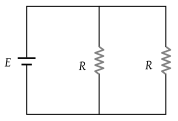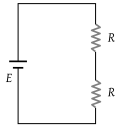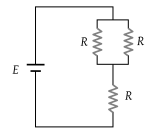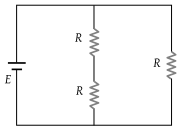NEET Questions Solved

Consider four circuits shown in the figure below. In which circuit power dissipated is greatest (Neglect the internal resistance of the power supply)

(1)(2)(3)(4)(1) $P=\frac{{V}^{2}}{{R}_{eq}};$ for P to be maximum ${R}_{eq}$ should be less. Hence option (1) is correct.

Difficulty Level:

• 52%
• 26%
• 11%
• 13%